# Template:LSU CT99CommonTheme2A

Referencing (equivalently, Version 1 of) the above-identified integral expression for the Gravitational Potential of an Axisymmetric Mass Distribution, Trova, Huré & Hersant (2012) offer the following assessment in §6 of their paper:

 "The important question we have tried to clarify concerns the possibility of converting the remaining double integral … into a line integral … this question remains open."

We also have wondered whether there is a possibility of converting the double integral in this Key Equation into a single (line) integral. This is a particularly challenging task when, as is the case with Version 1 of the expression, the integrand is couched in terms of cylindrical coordinates because the modulus of the elliptic integral is explicitly a function of both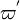$~\varpi^'$ and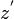$~z^'$.

We have realized that if we focus, instead, on Version 2 of the expression and associate the meridional-plane coordinates of the anchor ring with the coordinates of the location where the potential is being evaluated — that is, if we set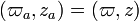$~(\varpi_a, z_a) = (\varpi,z)$ — the argument of the elliptic integral becomes,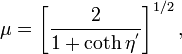$~\mu = \biggl[\frac{2}{1+\coth\eta^' }\biggr]^{1 / 2} \, ,$

while the integral expression for the gravitational potential becomes,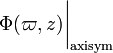$~\Phi(\varpi,z)\biggr|_\mathrm{axisym}$$~=$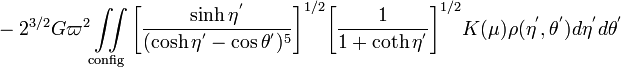$~ - 2^{3/2}G \varpi^2 \iint\limits_\mathrm{config} \biggl[\frac{ \sinh\eta^'}{(\cosh\eta^' - \cos\theta^')^5} \biggr]^{1 / 2} \biggl[\frac{1}{1+\coth\eta^' }\biggr]^{1 / 2} K(\mu) \rho(\eta^', \theta^') d\eta^' d\theta^'$$~=$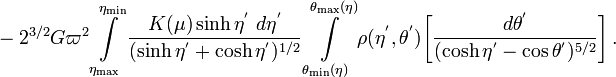$~- 2^{3 / 2} G \varpi^{2} \int\limits^{\eta_\mathrm{min}}_{\eta_\mathrm{max}} \frac{K(\mu) \sinh \eta^' ~d\eta^'}{( \sinh \eta^' +\cosh \eta^' )^{1 / 2}} \int\limits_{\theta_\mathrm{min}(\eta)}^{\theta_\mathrm{max}(\eta)} \rho(\eta^', \theta^') \biggl[ \frac{d\theta^'}{(\cosh\eta^' - \cos\theta^')^{5 / 2}} \biggr] \, .$

Notice that, by adopting this strategy, the argument of the elliptical integral is a function only of one coordinate — the toroidal coordinate system's radial coordinate,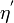$~\eta^'$. As result, the integral over the angular coordinate,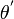$~\theta^'$, does not involve the elliptic integral function. Then — as is shown in an accompanying chapter titled, Attempt at Simplification — if the configuration's density is constant, the integral over the angular coordinate variable can be completed analytically. Hence, the task of evaluating the gravitational potential (both inside and outside) of a uniform-density, axisymmetric configuration having any surface shape has been reduced to a problem of carrying out a single, line integration. This provides an answer to the question posed by Trova, Huré & Hersant (2012).